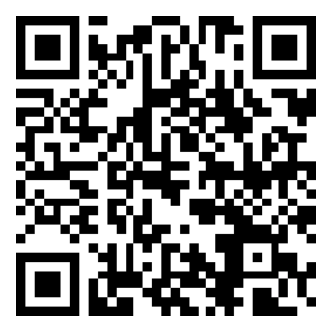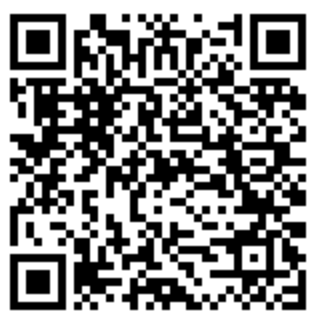# Calculating Percentage

I need to often be reminded of how to calculate a percentage.  The context here is that I want a progress bar in one of my programs.

To determine what percent a number is of a total number:
```(Progress So Far / Total Progress Units) * 100 = %
or
(Net Amount / Gross Amount) * 100 = %

eg. (12 steps / 30 steps) * 100 = 40%   ie. 12 steps of 30 is 40% progress
eg. (\$8 / \$10) * 100 = 80%              ie. \$8 of \$10 is 80%
```

To determine the percent (adjustment level) given the RRP and the price you're selling at:
```((Agreed Price - Usual Price) * 100) / Usual Price = %

eg. (\$10 - \$8) * 100 / \$8 = +25%       ie. selling an \$8 product at \$10 is an increase of 25%
eg. (\$8 - \$10) * 100 / \$10 = -20%      ie. selling a product at \$8 when it usually costs \$10 is a discount of 20%
eg. (\$7,562.50 - \$8,281.80) * 100 / \$8,281.80 = -8.69%
```

To determine the amount given the percentage and total amount:
```(Discount / 100) * Usual Price = Discounted Price

eg. (20 / 100) * \$80 = \$16       ie. 20% of \$80 is \$16
```

To determine the percent given two percentages:
```((Percentage 1 / 100) * (Percentage 2 / 100)) * 100 = %

eg. (50/100) * (40/100) = 0.5 x 0.4 = 0.2 * 100 = +20%       ie. 50% of a 40% sale is 20%
```

Opportunities won vs opportunities lost:
```Percentage = Won / (Won + Lost) * 100

eg. 50% = 1 / (1 + 1) * 100
```

Opportunities won vs total:
```Total = Won + Lost + Other
Percentage = Won / Total * 100

eg. 33.33% = 1 / (1 + 1 + 1) * 100
```

Markup Margin Percentage:
```( Sell - Cost ) / Cost * 100 = Markup Percent

eg. ( 75 - 50 ) / 50 * 100 = 50%
```

Gross Margin Percentage:
```( Sell - Cost ) = Gross Profit
( Sell - Cost ) / Sell * 100 = Gross Margin Percent

eg. ( 75 - 50 ) / 75 * 100 = 33.33%
```

### Accreditation### Donate & Support

If you like my content, and would like to support this sharing site, feel free to donate using a method below:

Paypal:Bitcoin:3QnhmaBX7LQSRsC9hh6Je9rGQKEGNQNfPb
© 2021 Joel Lipman .com. All Rights Reserved.